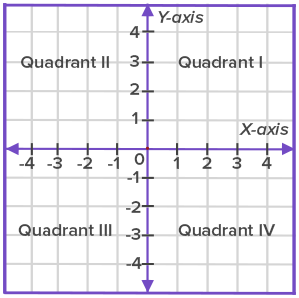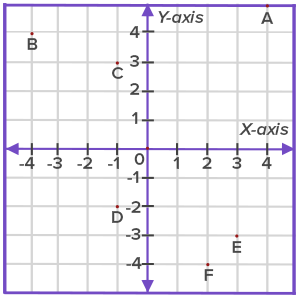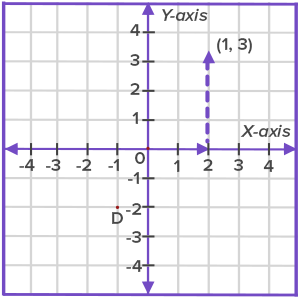# Coordinate Plane - Definition with Examples

The Complete K-5 Math Learning Program Built for Your Child

• 40 Million Kids

Loved by kids and parent worldwide

• 50,000 Schools

Trusted by teachers across schools

• Comprehensive Curriculum

Aligned to Common Core

What is Coordinate Plane?

A coordinate plane is a two-dimensional plane formed by the intersection of a vertical line called y-axis and a horizontal line called x-axis. These are perpendicular lines that intersect each other at zero, and this point is called the origin. The axes cut the coordinate plane into four equal sections, and each section is known as quadrant as shown below.The two-dimensional plane is called the Cartesian plane, or the coordinate plane and the axes are called the coordinate axes or x-axis and y-axis. The given plane has four equal divisions by origin called quadrants.  Quadrant 1, Quadrant 2, Quadrant 3 and Quadrant 4 show the division of the quadrant plane. The horizontal line towards the right of the origin (denoted by O) is positive x-axis. The horizontal line towards the left of the origin is negative x-axis. The vertical line above the origin is positive y-axis. The vertical line below the origin is negative y-axis.

The given conventions are followed to locate the coordinates of a point:

• The x-coordinate or abscissa of a point is its perpendicular distance from the y-axis measured along the x-axis.
• The y-coordinate or ordinate of a point is its perpendicular distance from the x-axis measured along the y-axis.

• In stating the coordinates of a point in the coordinate plane, the x-coordinate comes first, and then comes the y-coordinate. We place the coordinates in brackets as (x, y).

So, in the given graph, coordinates of A are (5, 6) as it is 5 units away from the origin on positive x-axis and 6 units away from the origin on the positive y-axis.

Can you tell the coordinates of B, D, E, and F?If a point is made somewhere on the graph as shown in the figure, how is its position represented?

 To plot the ordered pair (1, 3) in the coordinate plane:  First, plot the number 1 on the x-coordinate as it comes first in the ordered pair. Since it is a positive number, it should move 1 unit from the origin to the right. Next, plot the number 3 on the y-axis. As it is a positive number, so it would move 3 units up on the y-axis. The graphical representation of the ordered pair (1, 3) is shown in the figure.Fun Facts The coordinate plane was invented centuries ago. It was introduced by French mathematician René Descartes and thus, the system is sometimes called the Cartesian coordinate system.

Won Numerous Awards & Honors